# Physics - Electromagnetism - Sine waves and EM wave energyImage source:## Introduction

Hello it's a me again Drifter Programming! Today we continue with Electromagnetism to give more information around Sine waves (that we already covered a little bit in AC current) and also the energy of Electromagnetic waves. So, without further do, let's get straight into it!

## Sine waves

As we already saw in my Introduction article of AC current, sine waves are waves that are plotted as a graph, following the same periodic shape as the sine-function. Sine waves can be found in various things in nature, which include spring motion (that actually "slows down" and stops because of friction and air resistance), sound waves, radio waves etc. The electrical AC power that powers our houses and AC current from varying electrical voltages also follows such a shape and can be visualized easily using oscilloscopes.

Sine waves are measured by three main properties:

1. Amplitude - the maximum distance it ever reaches from zero
2. Frequency - The number of complete cycles per second
3. Period - The time taken to perform one complete cycle

Since the sine function varies in the range [-1, 1], the general sinusoidal waveform with Amplitude A, frequency f, period T and angular velocity ω is given by the mathematical expression: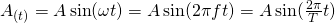As we already told last time, electromagnetic waves also follow such a shape when propagating in a vacuum of space or an actual medium. An electromagnetic wave is the combination of an electric and a magnetic wave, where both follow such a sinusoidal shape, but on a differenent 'axis', cause they are proportional to each other and also to the propagating direction. Including the wavelength and amplitude properties such waves are therefore represented by the following two eqautions:or the more general "wave equation":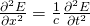(one for each wave - this one is for the electric "part")

## Electromagnetic wave energy density

As with most quantities that we talked about in Electromagnetism, Electromagnetic wave energy is more useful when talking about the density of it.

The ratio of the electric and magnetic field magnitudes is: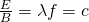which can be found using the previous two waveform-equation in "Maxwell" form, with Del, partial derivatives etc. and doing substitutions.

The total energy of a electromagnetic wave can therefore be described by one of the two wave magnitudes. When talking about densities the magnetic energy density is: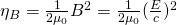Substituting 'c' with:we get: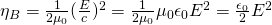Hmmm..this is the equation for electric energy density! This shows us that half the energy is electric and half the energy magnetic for electromagnetic fields. This means that the total energy density is:In terms of the magnetic field this can also be written as: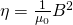All these eqautions work only for free space! (notice the constants that we are using)

It's of course much better to use this concept of energy, cause waves spread out in space and time, meaning that the energy fills a volume of space. That's why we sometimes also replace the energy with a energy per volume density, which looks as following: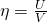where:

• U is the total potential energy of the two waves (covered in previous articles)
• V the total volume the wave covers

## EM wave power

When talking about Area we get into something called power density. The power density is defined as the power per area and so expressed as: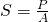The Power per unit area can be easily proven, starting out with the energy filling a volume dV, which is:where w is the energy stored per unit of volume given by ε0E^2 and dV = A c dt as the volume the wave passes in dt time.

The power per unit of area therefore comes out: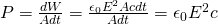Knowing that E = cB we have that the power is: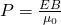The maximum/peak power is equal to: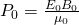The average power is half the peak power an given by: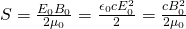where S is known as the intensity of the wave.

## Poynting vector

The previous expression was the traditional way of writing power density! The "real" equation is actually written in vector form as:where:

• S (power density), E (electric field) and B (magnetic field) are vectors.
• μ is the magnetic permeability (here given as μ0 for free space)

Vector S is given a oddly appropriate name "Poynting vector", in honor of John Poynting that discovered them using vector mathematics

Poynting vector is a quantity that describes the magnitude and direction of the flow of energy in electromagnetic waves. Power is the rate at which energy is being transported. For electromagnetic waves we think about it in terms of energy transportet per unit of area, cause it is easier in applications! This expression clearly shows us that the electric field and magnetic field are perpendicular to each other and that the vector product S is perpendicular to the plane that these two vectors (E and B) determine. This means that the Poynting vector also points in the direction of the wave propagation.

As already told in the previous "Heading" the average intensity of a wave is half the peak power. That's cause the average of the square of a sinusoidal function over a whole period is equal to '1/2'. Using the wave equation we can proof it very easily: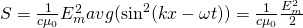Yes. I couldn't find a better way of giving the average :P

## Radiation pressure / Energy momentum

One last thing that is commonly used in applications considering EM wave energy is the so called radiation pressure and energy momentum (density).

The intensity (I) of the radiation is equal to the absolute, average value of the Poynting vector aka area power density. Replacing 'c' we can rewrite our previous equation as: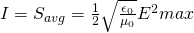(this "substitution" will be useful for formula simplifications)

We also say that EM waves carry a momentum P, that of course has some momentum density. The momentum carried by EM waves using the relativistic formula is known to be: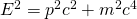From quantum mechanics on the other hand we know that the momentum of individual "massless" photon particles is: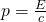From this equation we see that the momentum density for electromagnetic waves must be equal to the energy density divided by c. Since the energy density is u = ε0E^2 we have that: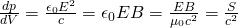We can further express the above momentum as the momentum transferred per unit time per unit of area, something that gives us:
As the momentum is a property of the field and not associated to the mass of a moving particle we can also define the phenomenon of radiation pressure. Using the average value of the Poynting vector S that an EM wave applies on an object and supposing no reflection and transmission, the radiation pressure on the object is:where dp is the infinitesimal change in momentum!

When all the incident electromagnetic waves are reflected by the object, the resulting radiation pressure is given by: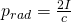### REFERENCES:

Mathematical equations that I had in this post where drawn using quicklatex!

## Previous articles of the Electromagnetism series

Here we only have the previous two "chapters" and the current one!

### Mutual and Self Induction:

Mutual Inductance -> Mutual Inductance, applications

Self Induction -> Self Induction, Lenz's law, Inductance of a Coil

Magnetic Energy Density -> Energy stored in a magnetic field (or inductor), Magnetic energy density, Coaxial Cable Inductance Example

R-L circuits -> R-L circuit energizing, de-energizing, Characteristic time constant

L-C circuits -> L-C circuit, oscillations, energy cases, applications

R-L-C circuits -> R-L-C circuit, oscillation, applications

Mutual and Self Induction exercises -> examples all around Mutual and Self Induction

### Alternating current:

Getting into Alternating current -> Phasor diagrams, Alternating current, Average (RMS) current and voltage, Differences, Advantages/Disadvantages

Electric Reactance in AC circuits -> Resistors, Inductor and Capacitor Reactance in AC circuits

Series R-L-C circuits Impedance -> Series R-L-C circuits, Phasor Diagrams, Impedance

Power in AC circuits -> DC Resistive circuit, Power in AC circuits (resistive, reactive), Power Factor

Resonance in Series R-L-C circuits -> Series R-L-C circuit, Series Resonance

Resonance in Parallel R-L-C circuits -> Parallel R-L-C circuit, Parallel Resonance

AC Transformers -> How they work, Configurations, Types, Voltage and Current Transformer

Alternating current exercises -> examples all around Alternating current

### Electromagnetic waves:

Electromagnetic Wave Introduction -> Electromagnetic waves, velocity, wave equation

## Final words | Next time

And this is actually it for today's post and I hope that you enjoyed it! Next time we will get into a very interesting topic: "Electromagnetic waves in matter!".Keep on drifting!

H2
H3
H4
3 columns
2 columns
1 column